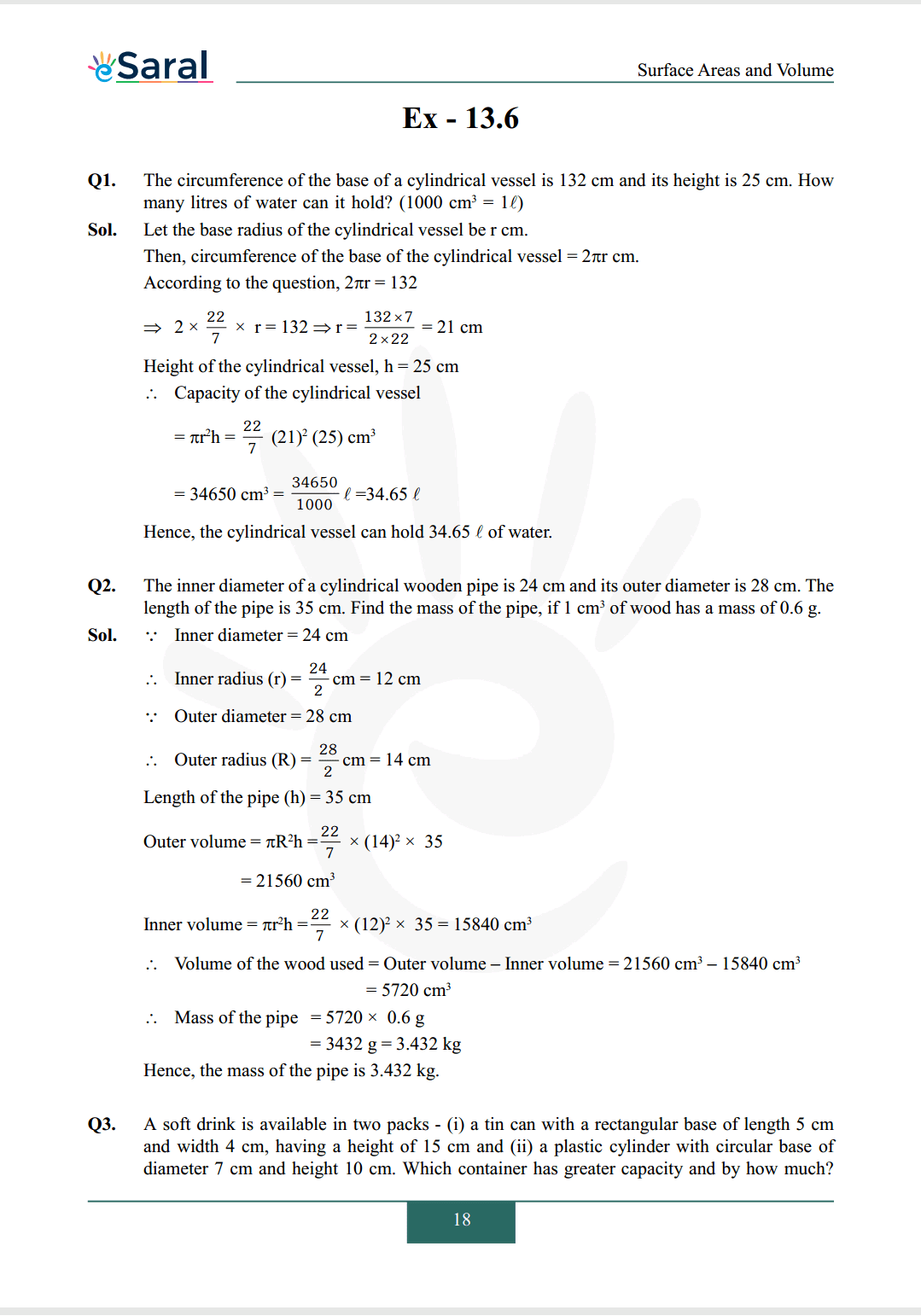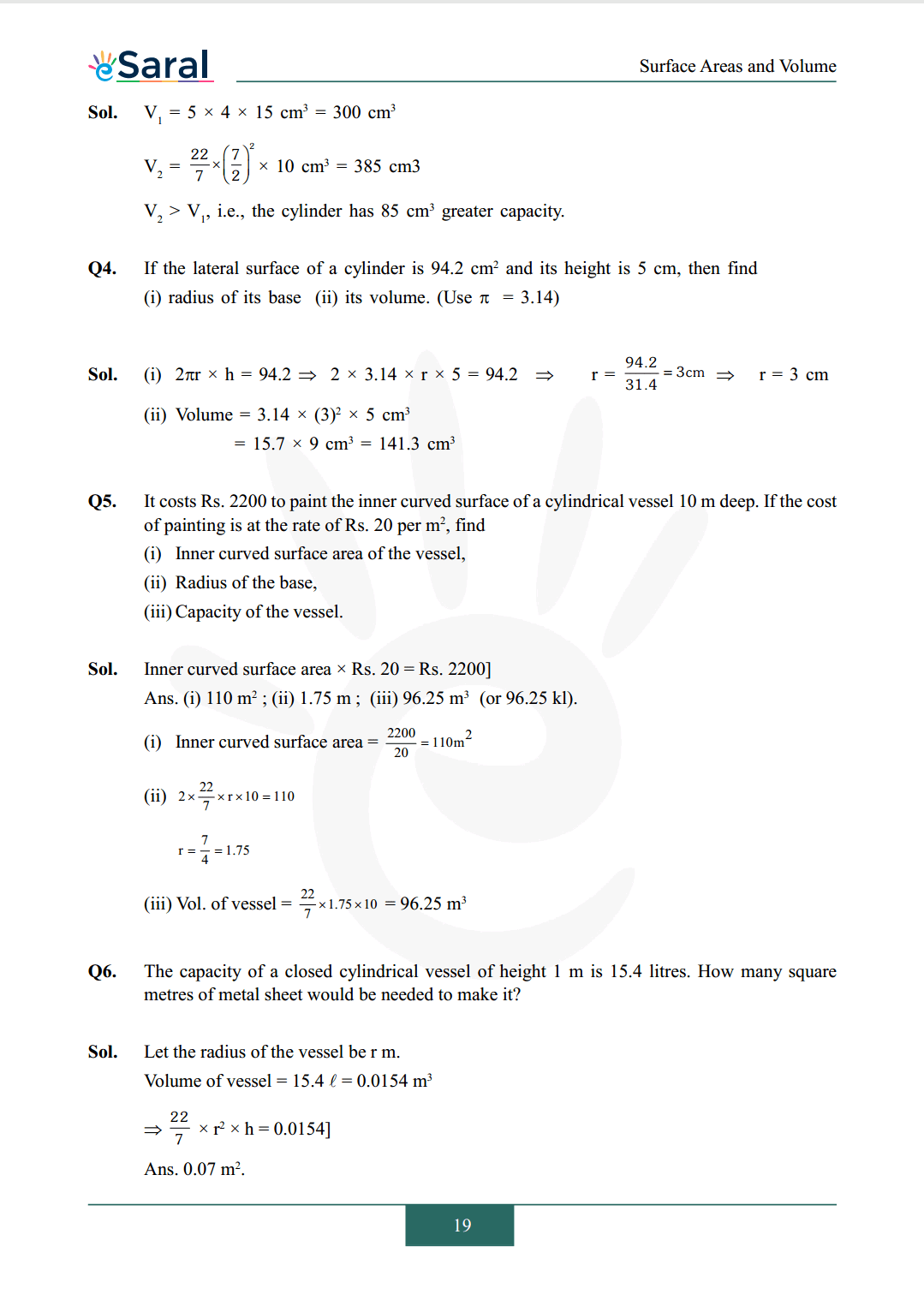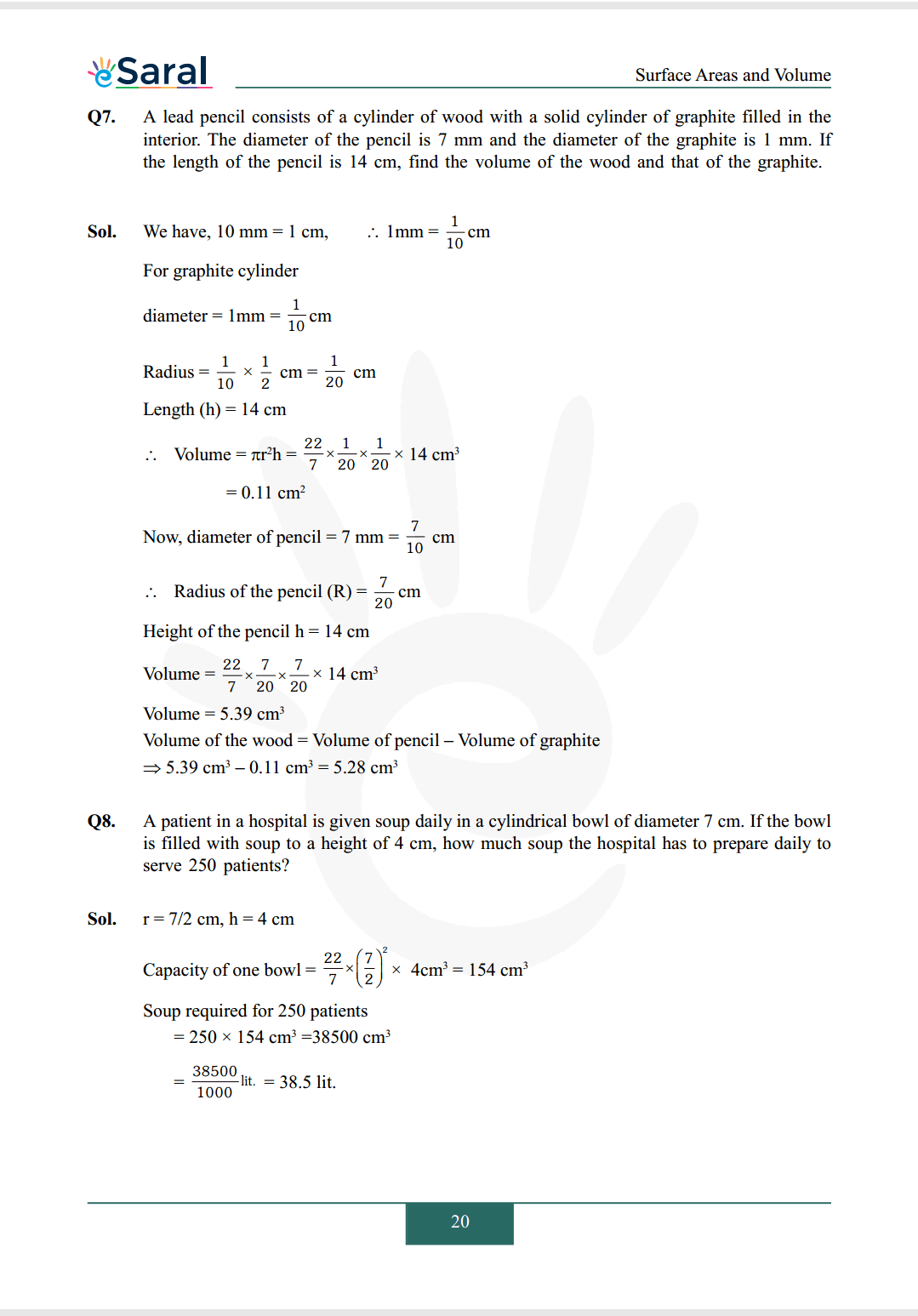Most Affordable JEE | NEET | 8,9,10 Preparation by Kota's Top IITian Doctor Faculties

# NCERT Solutions for Class 9 Maths chapter 13 Exercise 13.6 - Surface Areas and VolumesHey, are you a class 9 Student and Looking for Ways to Download NCERT Solutions for Class 9 Maths chapter 13 Exercise 13.6? If Yes then you are at the right place.

Here we have listed Class 9 maths chapter 13 exercise 13.6 solutions in PDF that is prepared by Kota’s top IITian’s Faculties by keeping Simplicity in mind.

If you want to score high in your class 9 Maths Exam then it is very important for you to have a good knowledge of all the important topics, so to learn and practice those topics you can use eSaral NCERT Solutions.

So, without wasting more time Let’s start.

### Download The PDF of NCERT Solutions for Class 9 Maths chapter 13 Exercise 13.6 "Surface Areas and Volumes"#### All Questions of Chapter 13 Exercise 13.6

Once you complete the chapter 13 then you can revise Ex. 13.6 by solving following questions

Q1. The circumference of the base of a cylindrical vessel is $132 \mathrm{~cm}$ and its height is $25 \mathrm{~cm}$. How many litres of water can it hold? $\left (1000 \mathrm{~cm} ^ {3} =1 \ell\right)$

Q2. The inner diameter of a cylindrical wooden pipe is $24 \mathrm{~cm}$ and its outer diameter is $28 \mathrm{~cm}$. The length of the pipe is $35 \mathrm{~cm}$. Find the mass of the pipe, if $1 \mathrm{~cm} ^ {3}$ of wood has a mass of $0.6 \mathrm{~g}$.

Q3. A soft drink is available in two packs - (i) a tin can with a rectangular base of length $5 \mathrm{~cm}$ and width $4 \mathrm{~cm}$, having a height of $15 \mathrm{~cm}$ and (ii) a plastic cylinder with circular base of diameter $7 \mathrm{~cm}$ and height $10 \mathrm{~cm}$. Which container has greater capacity and by how much?

Q4. If the lateral surface of a cylinder is $94.2 \mathrm{~cm} ^ {2}$ and its height is $5 \mathrm{~cm}$, then find
(ii) its volume. (Use $\pi=3.14$)

Q5. It costs Rs. 2200 to paint the inner curved surface of a cylindrical vessel $10 \mathrm{~m}$ deep. If the cost of painting is at the rate of Rs. 20 per $\mathrm{m}^ {2}$, find
(i) Inner curved surface area of the vessel,
(iii) Capacity of the vessel.

Q6. The capacity of a closed cylindrical vessel of height $1 \mathrm{~m}$ is $15.4$ litres. How many square metres of metal sheet would be needed to make it?

Q7. A lead pencil consists of a cylinder of wood with a solid cylinder of graphite filled in the interior. The diameter of the pencil is $7 \mathrm{~mm}$ and the diameter of the graphite is $1 \mathrm{~mm}$. If the length of the pencil is $14 \mathrm{~cm}$, find the volume of the wood and that of the graphite.

Q8. A patient in a hospital is given soup daily in a cylindrical bowl of diameter $7 \mathrm{~cm}$. If the bowl is filled with soup to a height of $4 \mathrm{~cm}$, how much soup the hospital has to prepare daily to serve 250 patients?

If you have any Confusion related to NCERT Solutions for Class 9 Maths chapter 13 Exercise 13.6 then feel free to ask in the comments section down below.

To watch Free Learning Videos on Class 9 by Kota’s top Faculties Install the eSaral App